# 3025. 连续正整数之和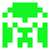2x+1=A
x=(A-1)/2-------------A 为奇数即可

x=(A-3)/3-----------A 能被 3 整除

x=(A-6)/4----------A-6 能被 4 整除
.5..：5x+10=A
x=(A-10)/5--------A-10 能被 5 整除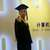n = 3时你算出来是2，实际上是1，加一个if(n == 3)就通过了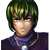from math import sqrt
T=int(input())
for I in range(T):
n=int(input())
count=0
for i in range(2, int(sqrt(2*n))+1, 2):
if n%i==i/2:
count+=1
for j in range(3, int(sqrt(2*n))+1, 2):
if n%j==0:
count+=1
print("case #%d:\n%d"%(I, count))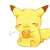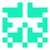//自己的一点想法…可能会有错误，且不太精准，欢迎指正。

example：

N = 9，part = 3 -> 3 4 5，offset = 3.

N = 21, part = 4 ->4,5,6,7 offset = 1. 故不成立。

bool couldbeput(const int test,const int part){
int result=test/part;
result=resultpart+part(part-1)/2;
int offset=test-result;
if(abs(offset)%abs(part)==0)
return true;
else
return false;

}
for(int i=2;i<(int)(sqrt(2*test+1)+1);i++)
if(couldbeput(test,i))